Next: About this document ... Up: lab_template Previous: lab_template

Subsections

# Vector Computations and Vector Functions

## Purpose

The purpose of this lab is to use Maple to introduce you to a number of useful commands for working with vectors, including some applications. The commands come from the Maple linalg and CalcP7 packages which must be loaded before any of its commands can be used.

## Getting Started

To assist you, there is a worksheet associated with this lab that you can copy into your home directory by going to your computer's start menu and choose run. In the run field type:

\\storage\academics\math\calclab


when you hit enter, you can then choose MA1023 and then choose the worksheet

Vector_start_B14.mw


Remember to immediately save it in your own home directory. Once you've copied and saved the worksheet, read through the background on the internet and the background of the worksheet before starting the exercises.

## Background

The commands below are some of the most basic vector commands. Some examples using these commands can be found in the Getting Started Worksheet. More examples can be found in the Help screens for each command.

innerprod
Computes the dot product (also known as the inner product) of two vectors.
crossprod
Computes the cross product of two vectors.
evalm
Evaluates expressions involving vectors (and matrices).
norm
Computes the norm, or length, of a vector.

## Angle between two vectors

If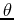is the angle between the vectorsand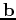, then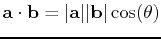.

## Vector Projection

The vector projection of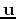ontoor the component ofin the direction ofcan be found using the following formula:The scalar component ofin the direction ofcan be found using the following formula: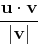## Area of a Triangle

The triangle created by connecting the terminal ends of the vectorsandin standard form has area =.

A unit vector perpendicular to the plane containing points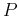,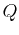and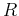is given by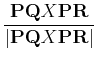.

## Exercises

1. Given the vectors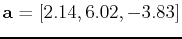and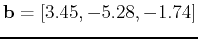, and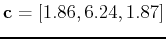, use Maple to compute the numeric value of the following expressions, if possible. For those that cannot be computed because they make no sense, please explain what is wrong.
1.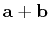2.3.4.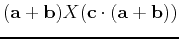2. Find the angle between each of the vectors above.

3. Find a vectorperpendicular tosuch that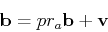whereand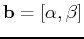. Then show thatandare perpendicular.

4. Find the equation of the line through the pointand parallel to the vector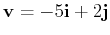. Plot the line and vector on the same graph.

5. For the vectors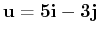and, find the vector projection ofontoand the scalar component ofin the direction of.

6. Find the area of the triangle with vertices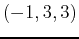,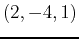and. (Hint: Shift the triangle to the origin by representing two of the sides of the triangles with vectors in standard form.) Also, find a unit vector perpendicular to the plane containing these three points.Next: About this document ... Up: lab_template Previous: lab_template
Dina J. Solitro-Rassias
2014-12-10